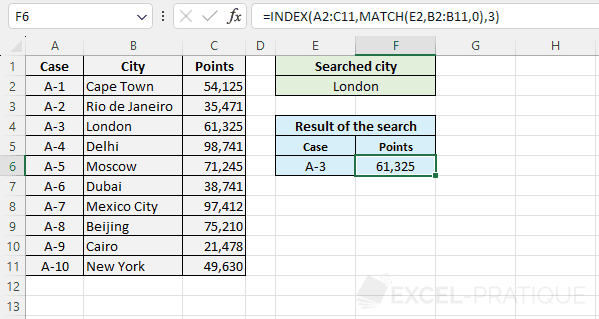# Excel Functions: INDEX + MATCH

The Excel function INDEX used with the function MATCH allows for value searching in an array.

Prerequisites:

Before proceeding, consult the tutorial on the function INDEX as well as that of the function MATCH.

To help you understand better, the example used here is a combination of the examples from the 2 indicated tutorials, so their reading is highly recommended.

## Combination INDEX + MATCH

If the MATCH function returns the row number:

`=INDEX(array, MATCH(lookup_value, lookup_array, 0), col_no)`

If the MATCH function returns the column number:

`=INDEX(array, row_no, MATCH(lookup_value, lookup_array, 0))`

## Example of use

The goal here is to find the case number as well as the number of points for the searched location. The user should be able to enter the city in the green part and then see the result of their search in the blue part: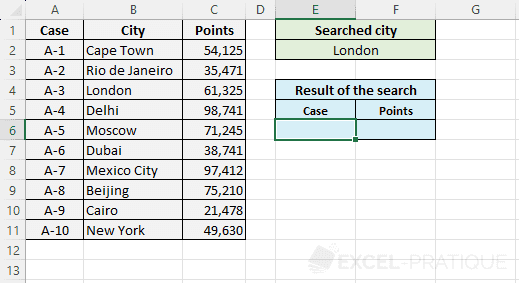First, select the INDEX function and enter:

• Array: the table with the data
• Row_num: don't enter anything for the moment (the MATCH function that will calculate the row number based on the search will be inserted here)
• Column_num: the column of the table that contains the data to be returned (in this example, the goal is to display the case number, so it is column 1)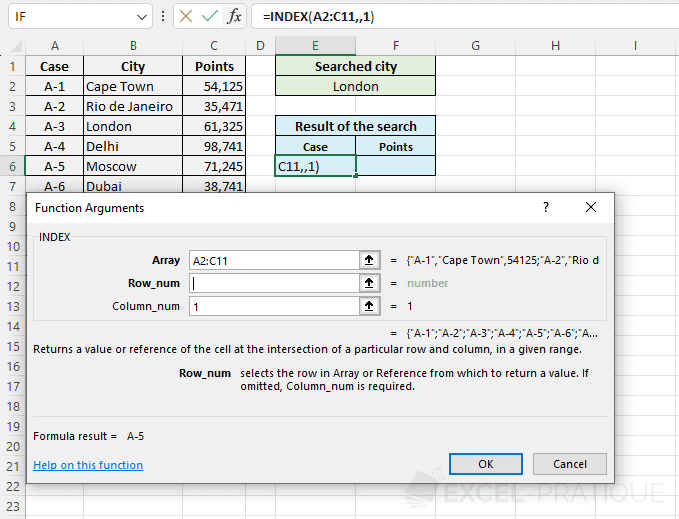Position yourself in Row_num and add the MATCH function: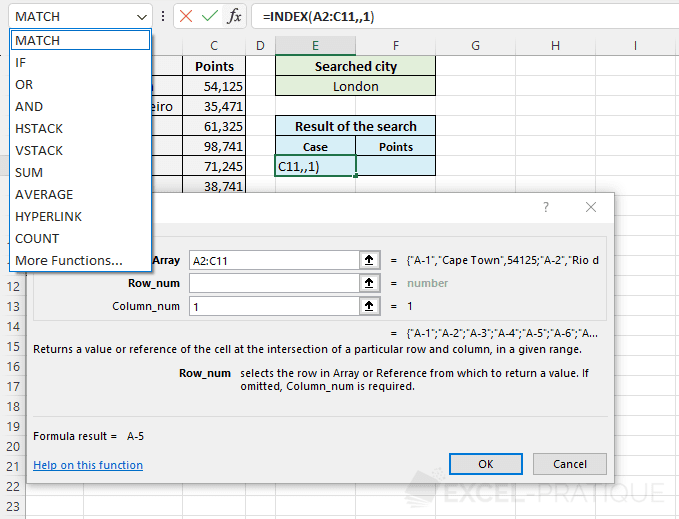For the MATCH function to return the row number of the city, enter:

• Lookup_value: the value to find the position of (here, the city)
• Lookup_array: the range of cells in which the function will search for the city's position
• Match_type: 0 to find the exact value
``=INDEX(A2:C11,MATCH(E2,B2:B11,0),1)``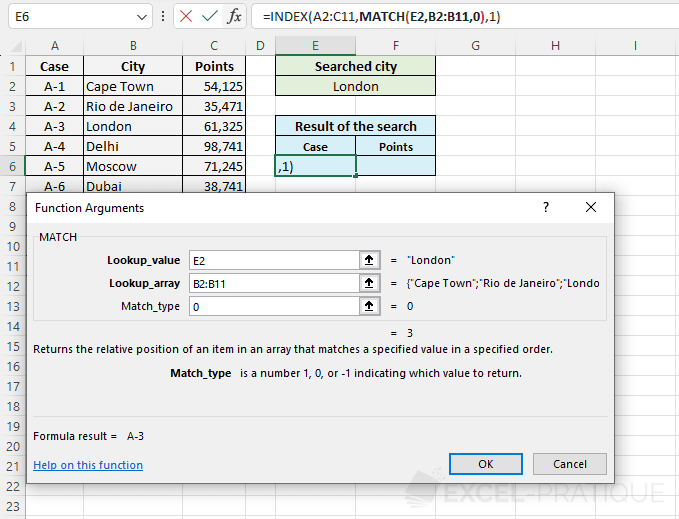The case number of the city is then displayed: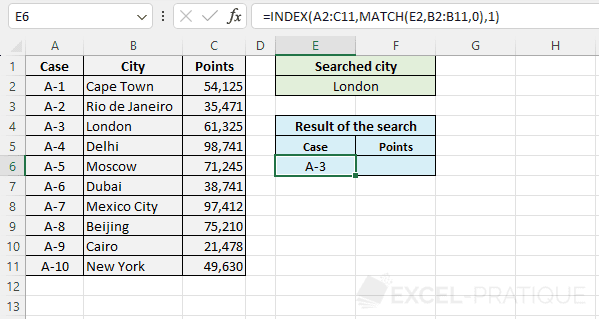To then display the points, just copy the formula and modify the column number (replace 1 with 3):